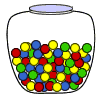# How To Calculate Sale Price and Discounts

## Learn About Calculating Percent Increase And Decrease With The Following Examples And Interactive Exercises.Problem: In a video store, a DVD that sells for \$15 is marked "10% off." What is the discount? What is the sale price of the DVD?

Analysis: Stores often sell goods for a discounted price. Typically, a store will discount an item by a percent of the original price. In this problem, an item that originally costs \$15 is being discounted by 10%. So "10% off" refers to the rate of discount. To solve this problem, we need a procedure.

Procedure:

1. The rate is usually given as a percent.
2. To find the discount, multiply the rate by the original price.
3. To find the sale price, subtract the discount from original price.

Now that we have a procedure, we can solve the problem above.Problem: In a video store, a DVD that sells for \$15 is marked, "10% off". What is the discount? What is the sale price of the DVD?

Solution: The rate is 10%.

The discount is: 0.10 x \$15.00 = \$1.50

The sale price is calculated as follows:

 \$15.00 original price -     1.50 - discount \$13.50 sale price

Answer: The discount is \$1.50 and the sale price is \$13.50.

Let's take a look at some more examples of calculating discount and sale price.Example 1: In a department store, a \$40 dress is marked, "Save 25%." What is the discount? What is the sale price of the dress?

Analysis: The phrase, "Save 25%," refers to the rate.

The original price of the dress is \$40.

Solution: The rate is 25%.

The discount is: 0.25 x \$40.00 = \$10.00

The sale price is calculated as follows:

 \$40.00 original price -    10.00 - discount \$30.00 sale price

Answer: The discount is \$10.00 and the sale price is \$30.00.Example 2: In a grocery store, a \$12 case of soda is labeled, "Get a 20% discount." What is the discount? What is the sale price of the case of soda?

Analysis: The phrase, "Get a 20% discount," refers to the rate.

Solution: The rate is 20%.

The discount is: 0.20 x \$12.00 = \$2.40

The sale price is calculated as follows:

 \$12.00 original price -    2.40 - discount \$ 9.60 sale price

Answer: The discount is \$2.40 and the sale price is \$9.60.Example 3: In a candy store, a \$5.00 jar of candy is labeled, "50% off." What is the discount? What is the sale price of the jar of candy?

Analysis: The phrase, "50% off," refers to the rate.

Solution: The rate is 50%.

The discount is: 0.50 x \$5.00 = \$2.50

The sale price is calculated as follows:

 \$5.00 original price -    2.50 - discount \$2.50 sale price

Answer: The discount is \$2.50 and the sale price is \$2.50.

In Example 3, note that the discount and the sale price are the same amount! Do you know what fraction is equal to 50%? Could you have done this problem using mental math? The phrase, "50% off," is the same as, "1/2 off". So using mental math, you would get that one-half of \$5.00 is \$2.50. Let's look at another example that uses a fraction.Example 4: A pizzeria has a coupon that reads, "Getoff a \$9.00 cheese pizza." What is the discount? What is the sale price of the cheese pizza?

Analysis: The phrase, "off," refers to the rate. It is expressed as a fraction.

Solution: The rate is given as the fraction.

The discount is:x \$9.00 = \$3.00

The sale price is calculated as follows:

 \$9.00 original price -    3.00 - discount \$6.00 sale price

Answer: The discount is \$3.00 and the sale price is \$6.00.

Once again, you could calculate the discount and sale price using mental math. Let's look at another way of calculating the sale price of an item. Below is a modified version of the problem from the top of this page.Example 5: In a video store, a DVD that sells for \$15 is marked, "10% off." What is the sale price of the DVD?

Solution: The rate is 10%. Thus, the customer is paying 90% for the DVD.

The sale price is: 0.90 x \$15.00 = \$13.50

Answer: The sale price is \$13.50.

Note that we calculated the sale price in the above problem, but we did not calculate the discount.

Summary: Stores often sell goods for a discounted price. Typically, a store will discount an item by a percent of the original price. The rate of discount is usually given as a percent, but may also be given as a fraction. The phrases used for discounted items include, "off," "Save 50%," and "Get a 20% discount."

Procedure:

1. To calculate the discount, multiply the rate by the original price.
2. To calculate the sale price, subtract the discount from original price.

### Exercises

Directions: Solve each problem below by entering a dollar amount with cents. For each exercise below, click once in the ANSWER BOX, type in your answer and then click ENTER. After you click ENTER, a message will appear in the RESULTS BOX to indicate whether your answer is correct or incorrect. To start over, click CLEAR.

 1.In a boutique, a \$14 scarf is marked, "20% off." What is the sale price of the scarf? ANSWER BOX:  \$  RESULTS BOX:
 2.In an electronics store, a \$75 iPod is labeled, "Save 15%." What is the sale price of the iPod? ANSWER BOX:  \$  RESULTS BOX:
 3.What is the discount for the iPod in Exercise 2? ANSWER BOX:  \$  RESULTS BOX:
 4.A \$30 shirt is marked, "Getoff." What is the sale price of the shirt? ANSWER BOX:  \$  RESULTS BOX:
 5.In a bicycle store, a \$500 bicycle is marked, "Get a 30% discount." What is the sale price of the bicycle? ANSWER BOX:  \$  RESULTS BOX: